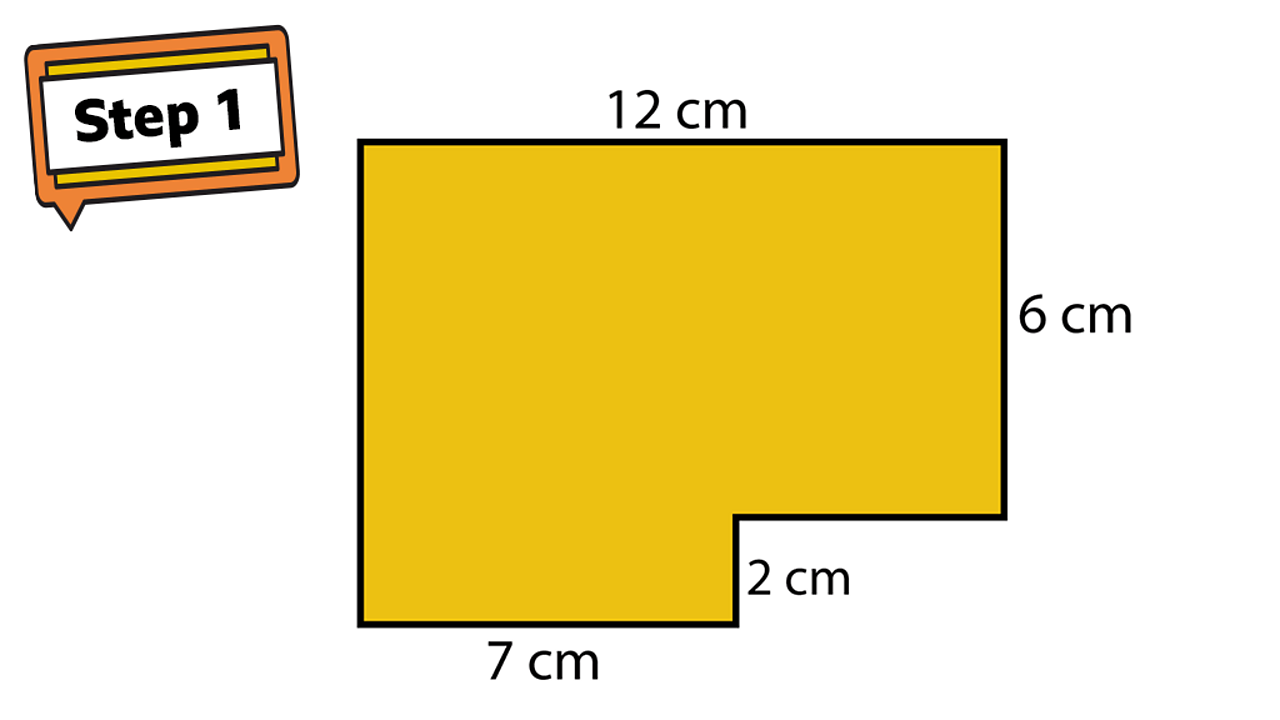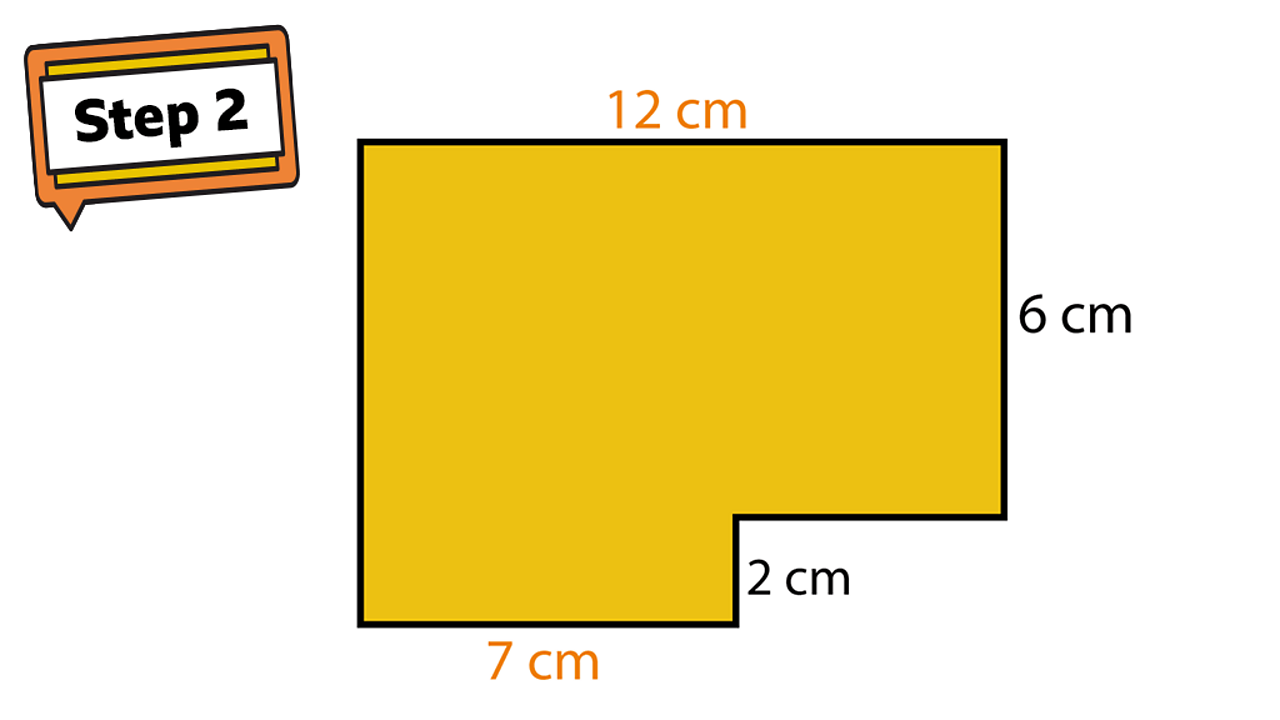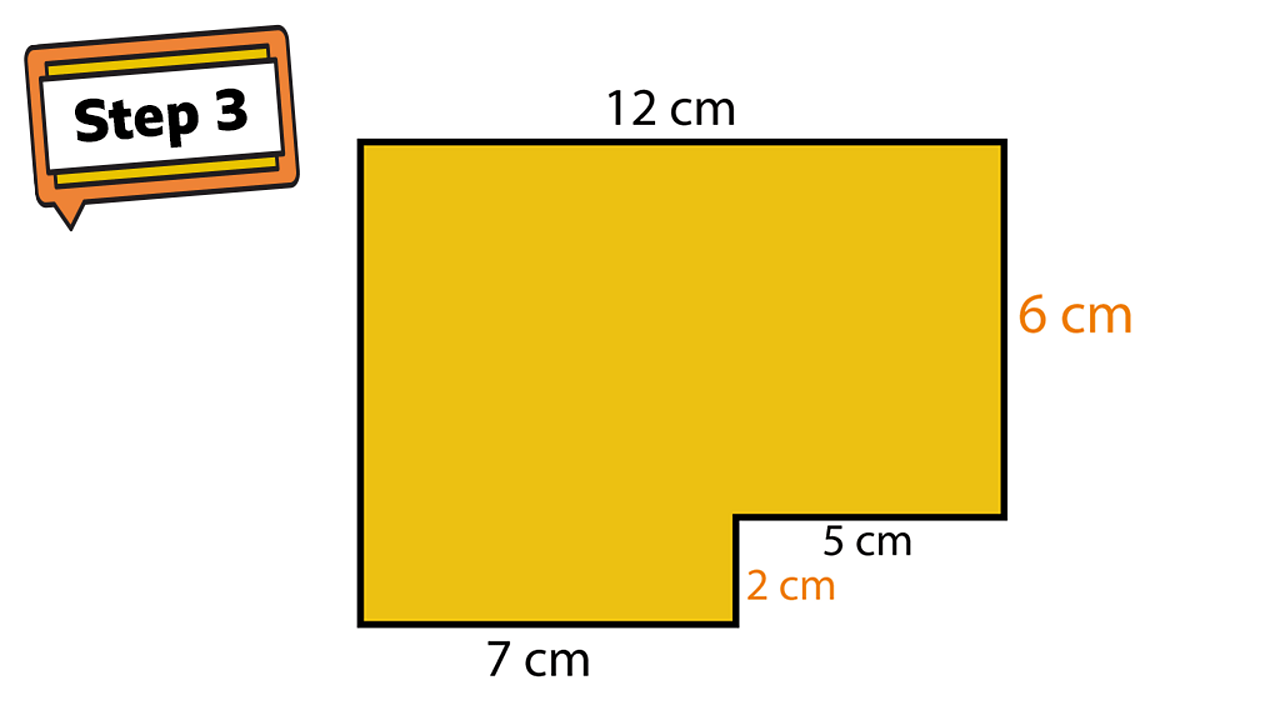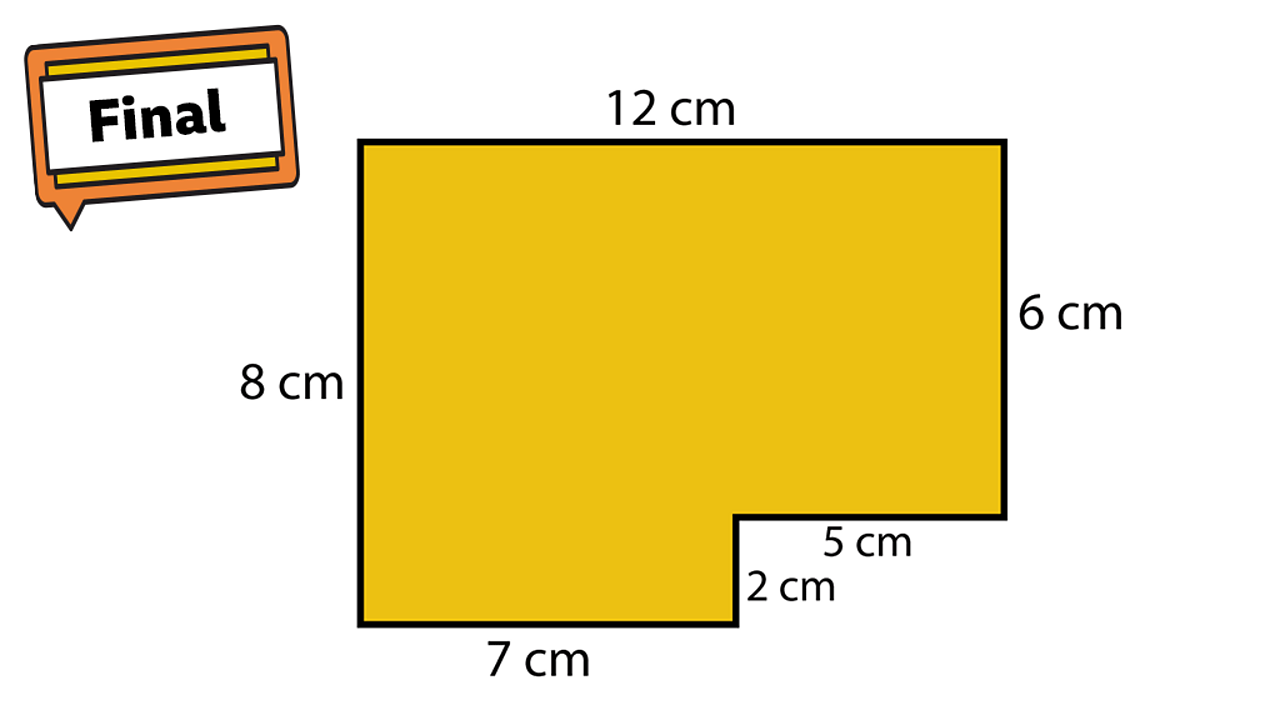# Finding the perimeter of a rectilinear shape

## Learning focus

Learn how to find the perimeter of a rectilinear shape.

This includes:

• a quiz
• a learning summary
• one video
• one activity

# Quiz

To get started, let's see how well you know this topic already. Take the quiz below to find out.

# Learn

A rectilinear shape is a shape that has straight sides and right angles. It can look like two rectangles that have been joined together.

This shape looks much more complicated than a rectangle, but the method of working out the perimeter is exactly the same.

Add together the lengths of all the sides to find it.

2 + 2 + 3 + 3 + 5 + 5 = 20

The perimeter is 20 cm

### Finding the unknown sides

Watch the video below from BBC Bitesize on finding the perimeter of a rectilinear shape when you don't know the length of every side.

## Example 1

Can you find the perimeter of this shape?

## Example 2

Work out the missing lengths of this shape to find the perimeter.To begin, let's look at the missing horizontal length.1 of 4The missing length needs to be added to 7 cm to create the longest length, 12 cm. 7 cm + ? = 12 cm. The missing length must be 5 cm.2 of 4Now let's look at the vertical sides. The longest length is missing, so you have to add 6 cm and 2 cm together to work it out. 6 cm + 2 cm = 8 cm.3 of 4Now you can work out the perimeter since you know the measurement of each side. 12 cm + 6 cm + 5 cm + 2 cm + 7 cm + 8 cm = 40 cm.4 of 4

# Practise

## Activity

Make a shape

Cut out 12 squares of paper of equal size or use 12 square sticky notes (you may have these from yesterday's activity). Arrange them into a rectilinear shape.

What is the perimeter of this shape?

Now rearrange the pieces of paper into a new shape. How many different shapes can you make and find the perimeter of?